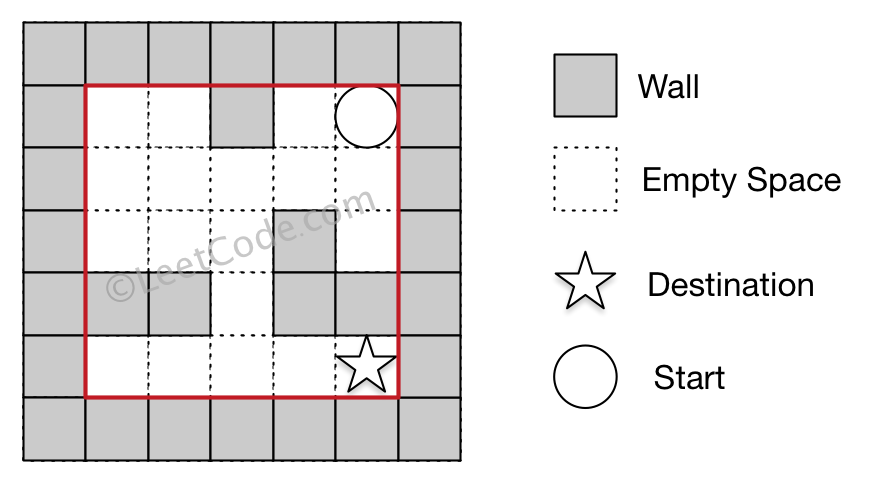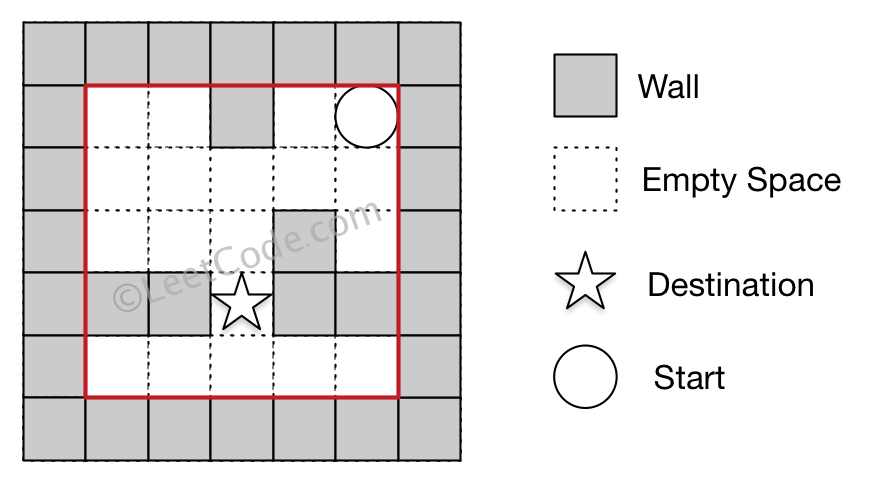##### Welcome to Subscribe On Youtube

Formatted question description: https://leetcode.ca/all/505.html

# 505. The Maze II

Medium

## Description

There is a ball in a maze with empty spaces and walls. The ball can go through empty spaces by rolling up, down, left or right, but it won’t stop rolling until hitting a wall. When the ball stops, it could choose the next direction.

Given the ball’s start position, the destination and the maze, find the shortest distance for the ball to stop at the destination. The distance is defined by the number of empty spaces traveled by the ball from the start position (excluded) to the destination (included). If the ball cannot stop at the destination, return -1.

The maze is represented by a binary 2D array. 1 means the wall and 0 means the empty space. You may assume that the borders of the maze are all walls. The start and destination coordinates are represented by row and column indexes.

Example 1:

Input 1: a maze represented by a 2D array

0 0 1 0 0
0 0 0 0 0
0 0 0 1 0
1 1 0 1 1
0 0 0 0 0

Input 2: start coordinate (rowStart, colStart) = (0, 4)
Input 3: destination coordinate (rowDest, colDest) = (4, 4)

Output: 12

Explanation: One shortest way is : left -> down -> left -> down -> right -> down -> right. The total distance is 1 + 1 + 3 + 1 + 2 + 2 + 2 = 12.Example 2:

Input 1: a maze represented by a 2D array

0 0 1 0 0
0 0 0 0 0
0 0 0 1 0
1 1 0 1 1
0 0 0 0 0

Input 2: start coordinate (rowStart, colStart) = (0, 4)
Input 3: destination coordinate (rowDest, colDest) = (3, 2)

Output: -1

Explanation: There is no way for the ball to stop at the destination.Note:

1. There is only one ball and one destination in the maze.
2. Both the ball and the destination exist on an empty space, and they will not be at the same position initially.
3. The given maze does not contain border (like the red rectangle in the example pictures), but you could assume the border of the maze are all walls.
4. The maze contains at least 2 empty spaces, and both the width and height of the maze won’t exceed 100.

## Solution

Initialize the distances to INFINITY for all positions except start, which has distance 0. Since the shortest distance is required, use priority queue during the search. Starting from start, each time obtain all possible positions the ball can reach and stop at, update the shortest distances for such positions, and then do further search from the new positions. If the ball can reach and stop at destination, update the shortest distance for destination. After all possible positions are visited, the shortest distances possible are also obtained. If the shortest distance at destination is not INFINITY, return the distance. Otherwise, the ball never reaches and stops at destination, return -1.

• class Solution {
public int shortestDistance(int[][] maze, int[] start, int[] destination) {
final int WALL = 1;
int rows = maze.length, columns = maze.length;
int[][] distances = new int[rows][columns];
for (int i = 0; i < rows; i++) {
for (int j = 0; j < columns; j++) {
if (maze[i][j] == WALL)
distances[i][j] = -1;
else
distances[i][j] = Integer.MAX_VALUE;
}
}
int shortestDistance = Integer.MAX_VALUE;
int[][] directions = { {-1, 0}, {1, 0}, {0, -1}, {0, 1} };
distances[start][start] = 0;
PriorityQueue<PositionDistance> priorityQueue = new PriorityQueue<PositionDistance>();
priorityQueue.offer(new PositionDistance(start, start, 0));
while (!priorityQueue.isEmpty()) {
PositionDistance positionDistance = priorityQueue.poll();
int row = positionDistance.getRow(), column = positionDistance.getColumn(), distance = positionDistance.getDistance();
if (distance > shortestDistance)
break;
for (int[] direction : directions) {
int moveCount = 0;
int deltaRow = direction, deltaColumn = direction;
int stopRow = row, stopColumn = column;
int curRow = row + deltaRow, curColumn = column + deltaColumn;
while (curRow >= 0 && curRow < rows && curColumn >= 0 && curColumn < columns && maze[curRow][curColumn] != WALL) {
moveCount++;
stopRow = curRow;
stopColumn = curColumn;
curRow += deltaRow;
curColumn += deltaColumn;
}
int newDistance = distance + moveCount;
if (newDistance < distances[stopRow][stopColumn]) {
distances[stopRow][stopColumn] = newDistance;
priorityQueue.offer(new PositionDistance(stopRow, stopColumn, newDistance));
}
}
shortestDistance = Math.min(shortestDistance, distances[destination][destination]);
}
if (distances[destination][destination] == Integer.MAX_VALUE)
return -1;
else
return shortestDistance;
}
}

class PositionDistance implements Comparable<PositionDistance> {
private int row;
private int column;
private int distance;

public PositionDistance() {

}

public PositionDistance(int row, int column, int distance) {
this.row = row;
this.column = column;
this.distance = distance;
}

public int getRow() {
return row;
}

public int getColumn() {
return column;
}

public int getDistance() {
return distance;
}

public int compareTo(PositionDistance positionDistance2) {
return this.distance - positionDistance2.distance;
}
}

• // OJ: https://leetcode.com/problems/the-maze-ii/
// Time: O(MN)
// Space: O(MN)
class Solution {
public:
int shortestDistance(vector<vector<int>>& A, vector<int>& S, vector<int>& E) {
int M = A.size(), N = A.size(), step = 0, dirs = { {0,1},{0,-1},{1,0},{-1,0} };
vector<vector<vector<bool>>> seen(M, vector<vector<bool>>(N, vector<bool>(4))); // (x, y, direction)
queue<array<int, 3>> q;
for (int i = 0; i < 4; ++i) {
seen[S][S][i] = true;
q.push({S, S, i});
}
while (q.size()) {
int cnt = q.size();
while (cnt--) {
auto [x, y, dir] = q.front();
q.pop();
auto &[dx, dy] = dirs[dir];
int nx = x + dx, ny = y + dy;
if (nx < 0 || nx >= M || ny < 0 || ny >= N || A[nx][ny]) { // The ball hits a wall. We can probe 4 directions
if (x == E && y == E) return step; // we can only check (x, y) if we are by a wall
for (int d = 0; d < 4; ++d) {
if (d == dir) continue;
auto &[dx, dy] = dirs[d];
int nx = x + dx, ny = y + dy;
if (nx < 0 || nx >= M || ny < 0 || ny >= N || A[nx][ny] || seen[nx][ny][d]) continue;
seen[nx][ny][d] = true;
q.push({nx, ny, d});
}
} else if (!seen[nx][ny][dir]) { // The ball doesn't hit a wall. We can only move in the same direction.
seen[nx][ny][dir] = true;
q.push({nx, ny, dir});
}
}
++step;
}
return -1;
}
};

• class Solution:
def shortestDistance(
self, maze: List[List[int]], start: List[int], destination: List[int]
) -> int:
m, n = len(maze), len(maze)
rs, cs = start
rd, cd = destination
dist = [[inf] * n for _ in range(m)]
dist[rs][cs] = 0
q = deque([(rs, cs)])
while q:
i, j = q.popleft()
for a, b in [[0, -1], [0, 1], [1, 0], [-1, 0]]:
x, y, step = i, j, dist[i][j]
while 0 <= x + a < m and 0 <= y + b < n and maze[x + a][y + b] == 0:
x, y, step = x + a, y + b, step + 1
if step < dist[x][y]:
dist[x][y] = step
q.append((x, y))
return -1 if dist[rd][cd] == inf else dist[rd][cd]

############

class Solution(object):
def shortestDistance(self, maze, ball, hole):
"""
:type maze: List[List[int]]
:type start: List[int]
:type destination: List[int]
:rtype: int
"""

def next(curr, maze):
height = len(maze)
width = len(maze)
directions = [(-1, 0), (1, 0), (0, -1), (0, 1)]
for di, dj in directions:
dist = 0
i, j = curr
while 0 <= i + di < height and 0 <= j + dj < width and maze[i + di][j + dj] != 1:
i += di
j += dj
dist += 1
yield (i, j), dist

heap = [(0, tuple(ball))]
visited = set()
hole = tuple(hole)
while heap:
dist, curr = heapq.heappop(heap)
if curr in visited:
continue
visited |= {curr}
if curr == hole:
return dist
for pos, incDist in next(curr, maze):
heapq.heappush(heap, (dist + incDist, pos))

return -1


• func shortestDistance(maze [][]int, start []int, destination []int) int {
m, n := len(maze), len(maze)
dist := make([][]int, m)
for i := range dist {
dist[i] = make([]int, n)
for j := range dist[i] {
dist[i][j] = math.MaxInt32
}
}
dist[start][start] = 0
q := [][]int{start}
dirs := []int{-1, 0, 1, 0, -1}
for len(q) > 0 {
i, j := q, q
q = q[1:]
for k := 0; k < 4; k++ {
x, y, step := i, j, dist[i][j]
a, b := dirs[k], dirs[k+1]
for x+a >= 0 && x+a < m && y+b >= 0 && y+b < n && maze[x+a][y+b] == 0 {
x, y, step = x+a, y+b, step+1
}
if step < dist[x][y] {
dist[x][y] = step
q = append(q, []int{x, y})
}
}
}
if dist[destination][destination] == math.MaxInt32 {
return -1
}
return dist[destination][destination]
}